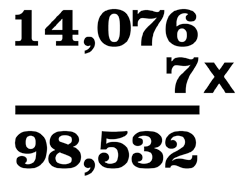Conundrums pay tribute to a singular digit – number 7

The required series of six primes in arithmetical progression is 7, 37, 67, 97, 127 and 157. In the second problem, the skeletal sum reconstructs to...

The required series of six primes in arithmetical progression is 7, 37, 67, 97, 127 and 157.

In the second problem, the skeletal sum reconstructs to formThis is the only fraction of this type known to established theory to equal one-seventh.

Try more puzzlers >>